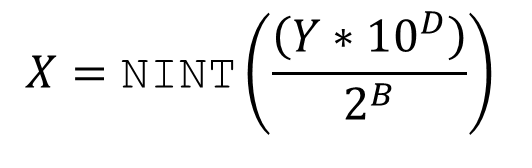# Binary Scaling of 2.5-km CONUS Gridded LAMP GRIB2 Files

The Localized Aviation Model Output Statistics Program (LAMP) is producing Gridded LAMP (GLMP) analyses and forecasts over the CONUS at 2.5-km grid resolution via NCEP's parallel production system. GLMP generates forecasts for the elements of temperature, dewpoint temperature, continuous ceiling height, and continuous visibility for projections 1-25 hours. Since each GRIB2 file contains several grids (one per projection hour) at a 2.5-km resolution, these GRIB2 files can be large. In order to reduce the GRIB2 file sizes, MDL will use binary scaling when packing 2.5-km NDGD data into GRIB2. Previously, MDL used decimal scaling which did not affect the precision of the forecast guidance. Decimal scaling simply multiplies the data by powers of 10 (i.e. any real value forecast will be packed as integer). The following equation describes the scaling used in GRIB2 packing:where X = packed value; Y = actual value; D = decimal scale factor; B = binary scale factor. The C function, NINT, allows the value to be rounded to the nearest integer, instead of truncating. As shown from the equation above, binary scaling is performing division arithmetic on the data which will introduce a loss of precision.

MDL began to use binary scaling with the 1200 UTC cycle on June 21, 2011 when packing data in GRIB2 format for the GLMP elements listed in the table below, with the addition of wind speed and wind direction analysis and forecast. This change is announced in TIN 15-xx (TBD; will be updated when available). Click on the element name to see animated GIF images comparing non binary scaling vs. binary scaling.

Element Original Precision Decimal Scale Factor Binary Scale Factor Precision After Scaling

Temperature Analysis

0.1 K

1

2

0.4 K

Temperature Error Analysis

0.1 K

1

2

0.4 K

Dewpoint Temperature Analysis

0.1 K

1

2

0.4 K

Dewpoint Temperature Error Analysis

0.1 K

1

2

0.4 K

Ceiling Height Analysis

1 M

0

3

8 M

Visibility Analysis

1 M

0

7

128 M

Wind Speed Analysis

0.1 m/s

1

2

0.4 m/s

Wind Gust Analysis

0.1 m/s

1

2

0.4 m/s

Wind Direction Analysis

1 degree

-1

0

10 degrees

Temperature Forecast

0.1 K

1

2

0.4 K

Dewpoint Temperature Forecast

0.1 K

1

2

0.4 K

Ceiling Height Forecast

1 M

0

3

8 M

Visibility Forecast

1 M

0

7

128 M

Wind Speed Forecast

0.1 m/s

1

2

0.4 m/s

Wind Gust Forecast

0.1 m/s

1

2

0.4 m/s

Wind Direction Forecast

1 degree

-1

0

10 degrees

The following elements do not have scaling applied:

• Sky Cover
• Ceiling Height Probabilities
• Visibility Probabilities
• Convection Probabilities
• Lightning Probabilities
• Convection Potentials
• Lightning Potentials

Using the scaling factors listed above, we were able to decrease file sizes between 21% - 26% of their original size for the temperature analysis and forecasts, temperature error analysis, dewpoint temperature analysis and forecasts, dewpoint temperature error analysis, and ceiling height analysis and forecasts.  The file sizes for the visibility analysis and forecasts were reduced by approximately 61% and 50%, respectively.